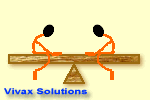# Mathematics

## Hard Algebraic Equations

#### Solving equationsAn equation is almost a sort of seesaw: you add something to the left, lose the balance and are forced to do the same to the right; you divide and multiply by something, once again, the same must be done to the other side; if you subtract something, there is no exception. Therefore, solving equation means, getting rid of everything around x by seesaw method.

E.g.1

2(x + 5) = 18
:- 2 => 2(x + 5) :- 2 = 18 :- 2
x + 5 = 9
- 5 => x + 5 - 5 = 9 - 5
x = 4

E.g.2

5(x - 2) = 2(x - 3)
5x - 10 = 2x - 6
+10 => 5x - 10 + 10 = 2x - 6 + 10
5x = 2x + 4
-2x => 5x - 2x = 2x - 2x + 4
3x = 4
:-3 => 3x / 3 = 4 /3
x = 1.3

E.g.3

4(x + 4) + 3(x -3) = 2(x -3) + 12
4x + 16 + 3x - 9 = 2x - 6 + 12
7x + 7 = 2x + 6
- 7 => 7x + 7 - 7 = 2x + 6 - 7
7x = 2x - 1
-2x => 7x - 2x = 2x - 2x -1
5x = -1
:-5 => 5x / 5 = -1 / 5
x = -0.2

E.g.4

(x + 5) / 4 = (x -3) / 2
X 4 => 4 X (x + 5) /4 = 4 X (x- 3) / 2
(x + 5) = 2 (x -3)
x + 5 = 2x - 6
- 5 => x +5 -5 = 2x - 6 - 5
x = 2x - 11
-2x => x - 2x = 2x - 2x -11
-x = -11
-1 X x = 11

E.g.5

3 + 2(x + 5) = 3 - (2x - 1)
3 + 2x + 10 = 3 -2x + 1
13 + 2x = 4 - 2x
-13 => 2x + 13 - 13 = 4 - 2x - 13
2x = -2x - 9
+2x => 2x + 2x = 2x - 2x - 9
4x = -9
:- 4 => 4x / 4 = -9 / 4
x = -2.25

#### Hard Equation Generator

With this simple programme, you can generate questions at random, along with answers - unlimited number of questions. Generate the question first, work out the solution and then check with the answer shown below the question.Maths is challenging; so is finding the right book. K A Stroud, in this book, cleverly managed to make all the major topics crystal clear with plenty of examples; popularity of the book speak for itself - 7th edition in print.

### Recommended - GCSE & iGCSEThis is the best book available for the new GCSE(9-1) specification and iGCSE: there are plenty of worked examples; a really good collection of problems for practising; every single topic is adequately covered; the topics are organized in a logical order.

### Recommended for A LevelThis is the best book that can be recommended for the new A Level - Edexcel board: it covers every single topic in detail;lots of worked examples; ample problems for practising; beautifully and clearly presented.